Logarithmic potential

(diff) ← Older revision | Latest revision (diff) | Newer revision → (diff)

A potential with the logarithmic kernel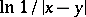, where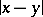is the distance between the pointsandof the Euclidean plane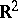, that is, a potential of the form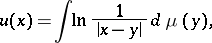(1)

where, generally, speaking, the integration is carried out with respect to an arbitrary Borel measureonwith compact support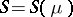. Physically one can assume that the logarithmic potential arises from the Newton potential of the forces of gravitation when the distribution of the attracting masses in the Euclidean spaceof points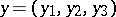does not depend, for example, on the coordinate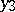. Of course the total mass is infinite, but if one performs a regularization of the resulting attracting force, which can be regarded as acting in the plane, consisting in discarding the infinite term, then the potential of the finite part ofwill invariably have the form (1) (see ). In contrast to the Newton kernel, the logarithmic kernel has a singularity not only as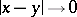, but also as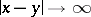, which causes some differences in the behaviour of the logarithmic potential as compared with the Newton potential. They occur mainly in the solution of exterior boundary value problems (see Exterior and interior boundary value problems). The main applications of the logarithmic potential occur in the solution of planar boundary value problems in potential theory (see also Boundary value problem, elliptic equations).

The main properties of the logarithmic potential are: 1) outside the supportof the measurethe logarithmic potential is a regular solution of the Laplace equation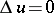, that is,is a harmonic function on the open set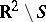, but is not regular at infinity, however; 2) if the measureis absolutely continuous, that is, the integral (1) takes the form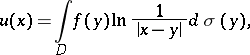(2)

whereis a finite domain,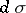is the area element ofand the densitybelongs to the class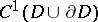, then the second derivatives ofare continuous inand satisfy the Poisson equation.

If the integral in (2) extends along a closed Lyapunov curve(see Lyapunov surfaces and curves), that is,(3)

one talks of the logarithmic potential of a single (or simple) layer, distributed on. If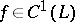, then the logarithmic potential of the single layer (3) is continuous everywhere in. Its normal derivative has limits from the inside and the outside of, respectively: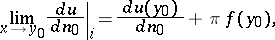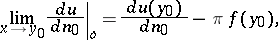where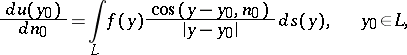(4)

is the so-called direct value of the normal derivative of the logarithmic potential of a single layer andis the angle between the vector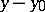and the outward normal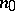toat the point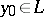. The integral (4) is continuous on.

The logarithmic potential of a double layer has the form(5)

whereis the outward normal toat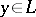. If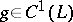, then the logarithmic potential of the double layer (5) is a regular harmonic function inside and outsideand has normal (non-angular) limits from the inside and the outside of, respectively: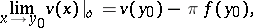whereis the direct value of the logarithmic potential of the double layer at the point. The normal derivative of the logarithmic potential of a double layer is continuous under transition through.

The listed boundary properties of the logarithmic potential of a simple and a double layer are completely analogous to the corresponding properties of the Newton potential (see also Potential theory). From (5) it is obvious that the logarithmic potential of a double layer is a harmonic function that is regular at infinity.

The logarithmic potential is also directly connected with boundary value problems of analytic function theory, since an integral of Cauchy type can be expressed in terms of the logarithmic potential of a single and a double layer (see ).

How to Cite This Entry:
Logarithmic potential. Encyclopedia of Mathematics. URL: http://encyclopediaofmath.org/index.php?title=Logarithmic_potential&oldid=11977
This article was adapted from an original article by E.D. Solomentsev (originator), which appeared in Encyclopedia of Mathematics - ISBN 1402006098. See original article